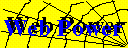# Power Analysis for ANOVA Designs

This online application has been retired. The web page remains here only for historical purposes.
See the Other links below for more modern alternatives.

This form runs a SAS program that calculates power or sample size needed to attain a given power for one effect in a factorial ANOVA design. The program is based on specifying Effect Size in terms of the range of treatment means, and calculating the minimum power, or maximum required sample size.

### Power Form

• Number of levels (a) of effect 'A'
• Total number of levels (b) of all other factors crossed with effect 'A'.
• Error level (alpha) for which you want power or sample size calculated
• Effect size(s) for which you want power or sample size calculated
• Output: Power table Sample-size table&The calculations are performed using a SAS macro program, fpower.sas, which is somewhat more general than presented here. The web interface is written as a perl script, power.pl. This script uses the cgi-utils.pl by Lincoln Stein.